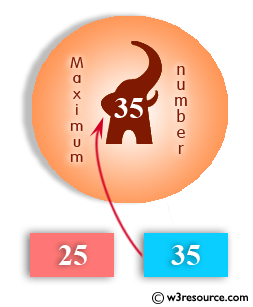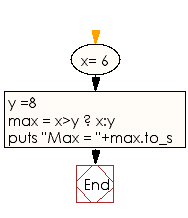﻿ Ruby Basic exercises: Find the maximum of two numbers - w3resource# Ruby Basic Exercises: Find the maximum of two numbers

## Ruby Basic: Exercise-18 with Solution

Write a Ruby program to find the maximum of two numbers.Ruby Code:

``````x= 6
y =8
max = x>y ? x:y
puts "Max = "+max.to_s
``````

Output:

```Max = 8
```

Flowchart:Ruby Code Editor: